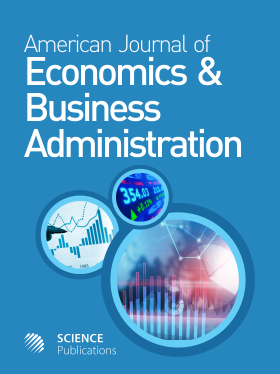Frequency: Continuous
ISSN: 1945-5488 (Print)
ISSN: 1945-5496 (Online)
Research Article Open Access

# Accelerators in Macroeconomics: Comparison of Discrete and Continuous Approaches

Valentina V. Tarasova1 and Vasily E. Tarasov1
• 1 Lomonosov Moscow State University, Russia

## Abstract

We prove that the standard discrete-time accelerator equation cannot be considered as an exact discrete analog of the continuous-time accelerator equation. This leads to fact that the standard discrete-time macroeconomic models cannot be considered as exact discretization of the corresponding continuous-time models. As a result, the equations of the continuous and standard discrete models have different solutions and can predict the different behavior of the economy. In this study, we propose a self-consistent discrete-time description of the economic accelerators that is based on the exact finite differences. For discrete-time approach, the model equations with exact differences have the same solutions as the corresponding continuous-time models and these discrete and continuous models describe the same behavior of the economy. Using the Harrod-Domar growth model as an example, we show that equations of the continuous-time model and the suggested exact discrete model have the same solutions and these models predict the same behavior of the economy.

American Journal of Economics and Business Administration
Volume 9 No. 3, 2017, 47-55

DOI:

Submitted On: 17 July 2017 Published On: 10 November 2017

How to Cite: Tarasova, V. V. & Tarasov, V. E. (2017). Accelerators in Macroeconomics: Comparison of Discrete and Continuous Approaches. American Journal of Economics and Business Administration, 9(3), 47-55. https://doi.org/10.3844/ajebasp.2017.47.55

• 2,922 Views